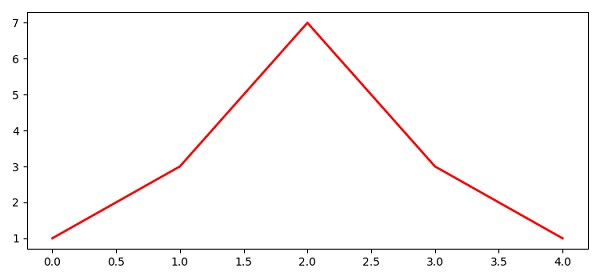# How to make pylab.savefig() save image for 'maximized' window instead of default size in Matplotlib?

To save image for maximized window instead of default size, we can use the following Steps −

• Create figure with figsize=(7.50, 3.50), using the figure() method.

• Plot the lines using the plot() method with list, color=”red”, and linewidth=2.

• Save the figure using the savefig() method.

• To display the figure, use the show() method.

## Example

from matplotlib import pyplot as plt
plt.rcParams["figure.figsize"] = [7.50, 3.50]
plt.rcParams["figure.autolayout"] = True
fig = plt.figure()
plt.plot([1, 3, 7, 3, 1], c="red", lw=2)
plt.savefig("full_image.png")
plt.show()

## Output